ANGSD: Analysis of next generation Sequencing Data

Latest tar.gz version is (0.936/0.937 on github), see Change_log for changes, and download it here.

# Error estimation method

The estimated rates can roughly be intrepreted as relative error rates. That is excess of errors in your sample compare to the error in the perfect indviduals. The idea is the your sample and the perfect individuals should have the same expected number of derived alleles and the extra derived alleles in you sample are due to the excess errorr. For each individual we sample a single base from the reads at each position. We use only positions were there are coverage for both the chimp, the sample and the perfect man. The overall error rate is obtained from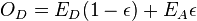$O_D = E_D(1-\epsilon) + E_A\epsilon$

were

•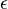$\epsilon$ is the error rate
•$O_D$ is the observed number of derived alleles in the sample
•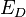$E_D$ is the expected number of derived alleles which is obtained from the observed derived alleles from the perfect man
•$E_A$ is the expected number of ancestral alleles which is obtained from the perfect man

For the type specific error rates are obtained from maximizing the likehood$p(H=h|C=c) = p(H=h|C=c,no error) \left(1-\sum_{h'\ne h} e_{h->h'} \right) + \left(\sum_{h'\ne h}p(H=h'|C=c,no error)e_{h'->h}\right)$

where

•$h$ is the allele of you sample
•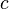$c$ is the allele of the chimp
•$e_{a->b}$ is the error rate for base a to base b
•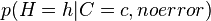$p(H=h|C=c,no error)$ are obtained from the perfect man assuming that the perfect man has no errors.